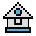Home

# IF ... THEN ... ELSE Statement

#### Purpose:

To make a decision regarding program flow based on the result returned by an expression.

#### Syntax:

```IF expression[,] THEN statement(s)[,][ELSE statement(s)]
IF expression[,] GOTO line number[[,] ELSE statement(s)]```

If the result of expression is nonzero (logical true), the THEN or GOTO line number is executed.

If the result of expression is zero (false), the THEN or GOTO line number is ignored and the ELSE line number, if present, is executed. Otherwise, execution continues with the next executable statement. A comma is allowed before THEN and ELSE.

THEN and ELSE may be followed by either a line number for branching, or one or more statements to be executed.

GOTO is always followed by a line number.

If the statement does not contain the same number of ELSE's and THEN's line number, each ELSE is matched with the closest unmatched THEN. For example:

`IF A=B THEN IF B=C THEN PRINT "A=C" ELSE PRINT "A < > C"`

will not print "A < > C" when A < > B.

If an IF...THEN statement is followed by a line number in the direct mode, an "Undefined line number" error results, unless a statement with the specified line number was previously entered in the indirect mode.

Because IF ..THEN...ELSE is all one statement, the ELSE clause cannot be on a separate line. It must be all on one line.

#### Nesting of IF Statements

IF...THEN...ELSE statements may be nested. Nesting is limited only by the length of the line. For example, the following is a legal statement:

```100 IF X > Y THEN PRINT "GREATER" ELSE IF Y > X THEN&
110 PRINT "LESS THAN"
200 ELSE PRINT "EQUAL"```

#### Testing Equality

When using IF to test equality for a value that is the result of a floating-point computation, remember that the internal representation of the value may not be exact. Therefore, test against the range over which the accuracy of the value may vary.

For example, to test a computed variable A against the value 1.0, use the following statement:

`IF ABS (A-1.0)<1.0E-6 THEN ...`

This test returns true if the value of A is 1.0 with a relative error of less than 1.0E-6.

#### Examples:

The following statement gets record number N, if N is not zero.

`200 IF N THEN GET#1, N`

In the following example, a test determines if N is greater than 10 and less than 20. If N is within this range, DB is calculated and execution branches to line 300. If N is not within this range, execution continues with line 110.

```100 IF(N<20) and (N>10) THEN DB=1979-1: GOTO 300
110 PRINT "OUT OF RANGE"```

The next statement causes printed output to go either to the terminal or the line printer, depending on the value of a variable (IOFLAG). If IOFLAG is zero, output goes to the line printer; otherwise, output goes to the terminal.

`210 IF IOFLAG THEN PRINT A\$ ELSE LPRINT A\$`